# Estimation of Ultimate Bearing Capacity of Foundation Soil

This sheet estimates the ultimate bearing capacity of a shallow foundation subjected to low to moderate loading conditions.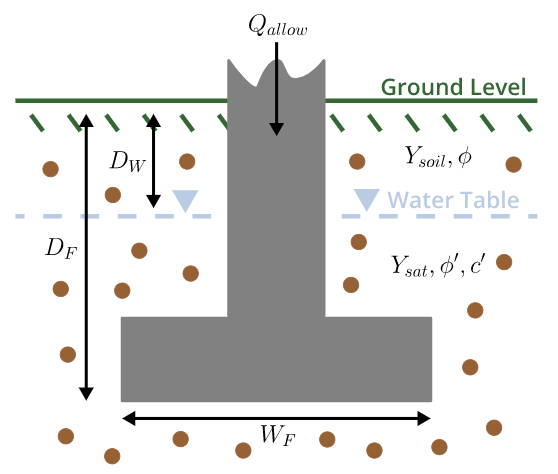## General Notes

• This sheet should be used only to estimate the bearing capacity of shallow foundation (e.g. column footing, mat-slab foundations, slab-on-grade foundations, pad foundations, rubble trench foundations and earthbag foundations).
• The ultimate bearing capacity of shallow foundation is the maximum load per unit area in foundation soil at which the shear failure occurred in the foundation.
• Meyerhof's bearing capacity equation has been used for estimation of bearing capacity of shallow foundation.
• Weight of the foundation is ignored in entire calculator.
• Effect of water table should be considered for the sites where permanent or seasonal water table is located.

## Basics of Meyerhof's Bearing Capacity Equation

• The ClearCalcs calculator will calculate the following factors based on the user inputs.
• Bearing Capacity Factors (Based on the angle of internal friction of foundation soil).
• Shape Factors (Based on the foundation geometry & angle of internal friction of foundation soil).
• Depth Factors (Based on the foundation width and depth).
• Inclination Factors (Based on the angle of load inclination on foundation)
• The effective stress at the bottom of foundation and the ultimate bearing capacity of shallow foundation then will be calculated.

## Input Required from The Users

#### Geometrical Details of Foundation

• The user needs to provide foundation depth, length and width as shown in the image below.
• It should be noted herein that larger foundation dimension should be entered as footing length and smaller foundation dimension should be entered as footing width.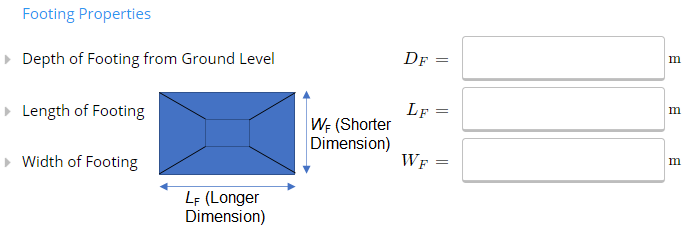#### Properties of Foundation Soil

• The user needs to provide foundation soil properties as shown in the image below.
• The angle of internal friction, cohesion and unit weight of foundation soil - This could be entered based on the available geotechnical interpretative report of the site. The users could also use our other Geotech templates (e.g., L type retaining wall, Gravity RW Masonry Blocks design templates) to estimate the properties of foundation soil.
• The Angle of load inclination is the angle at which the load is getting transferred to the foundation. In the case of vertical loads the angle of load inclination should be entered as 0.

##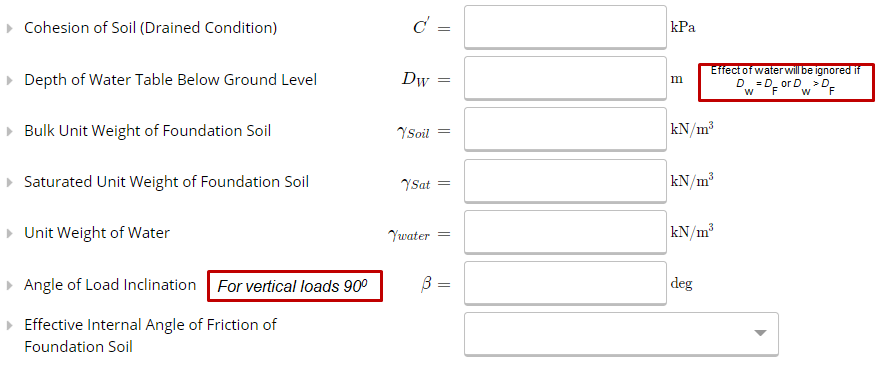Calculation of Ultimate Bearing Capacity of Foundation Soil

Once the key geometrical and foundation soil properties are entered the ClearCalcs bearing capacity calculator calculates the effective stress (q) at the foundation base and ultimate bearing capacity (qu) of foundation soil using the Meyerhof's equation (shown in the image below).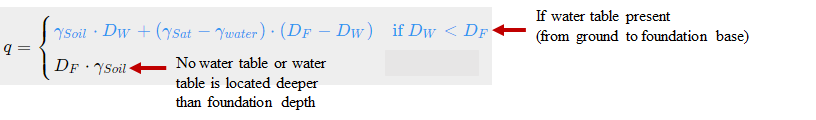### Calculation of Maximum Allowable Load Based on The Ultimate Bearing Capacity

The maximum allowable load on a given foundation base area could be calculated by entering a user defined factor of safety as shown below.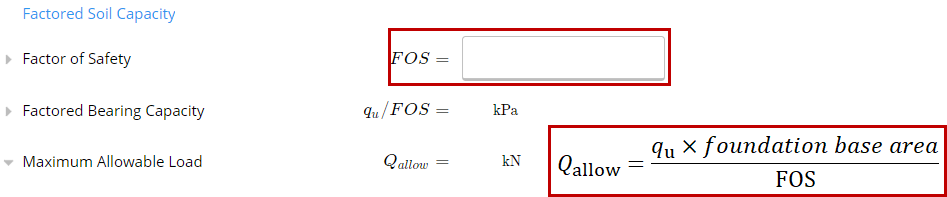## Design Example (Example 3.2, B. M. Das, 2011- Principles of Foundation Engineering)

A square foundation is 2 m x 2 m in plan. The soil supporting the foundation has a friction angle of 25 deg and effective cohesion of 20 kPa. The unit weight of soil is 16.5 kN/m3. Determine the allowable gross load on the foundation with a factor of safety (FS) of 3. Assume that the depth of the foundation (DF) is 1.5 m and that general shear failure occurs in the soil.

1. Input from the user - Footing Geometry: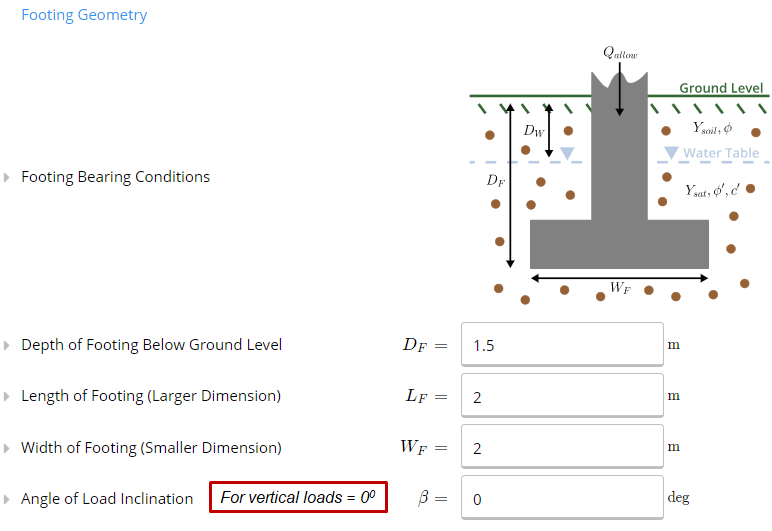2. Input from the user - Base soil properties: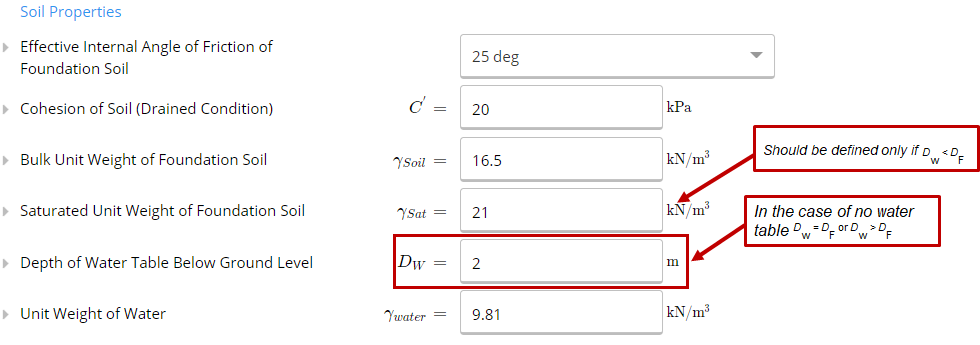3. The bearing capacity parameters is then calculated based on the friction angle of base soil, foundation dimensions and angle of load inclination.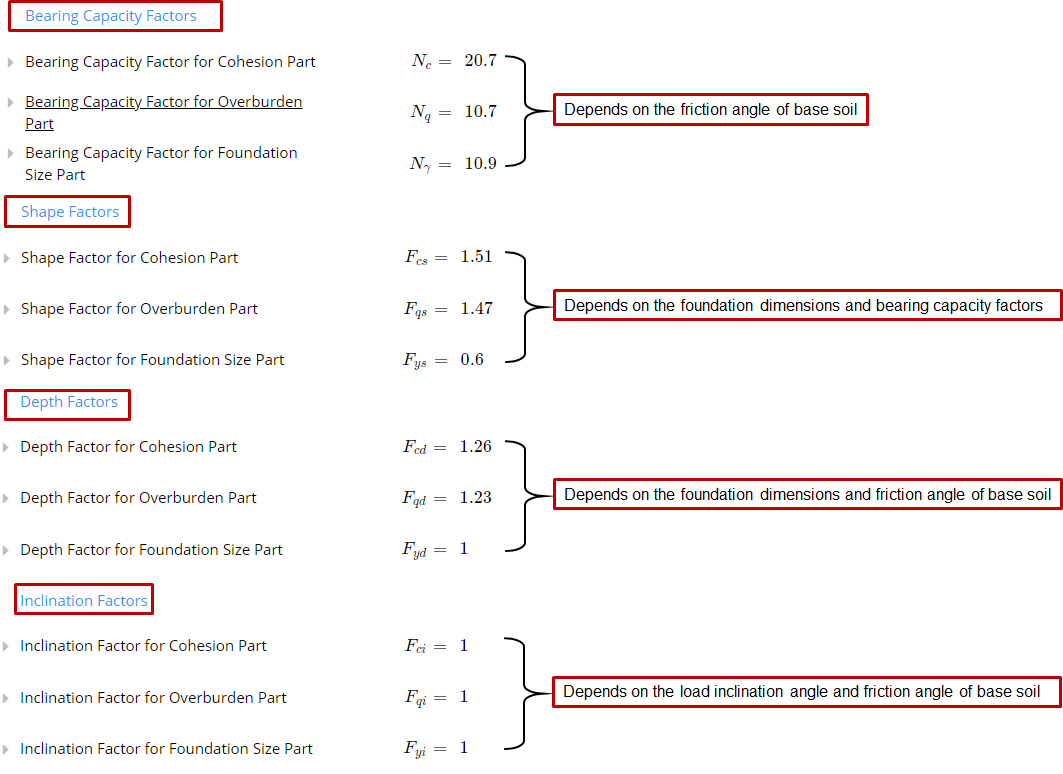4. Effective stress at foundation base and bearing capacity of foundation is then calculated as shown below: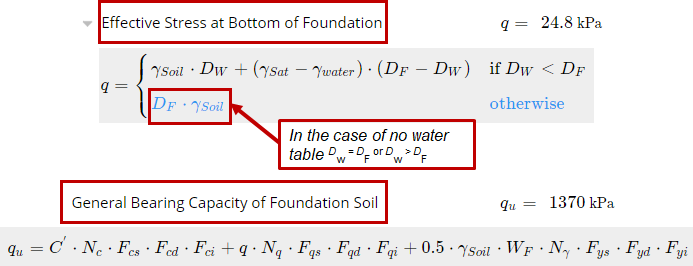5.    The allowable load at foundation will then be calculated using the user defined factor of safety and foundation base area.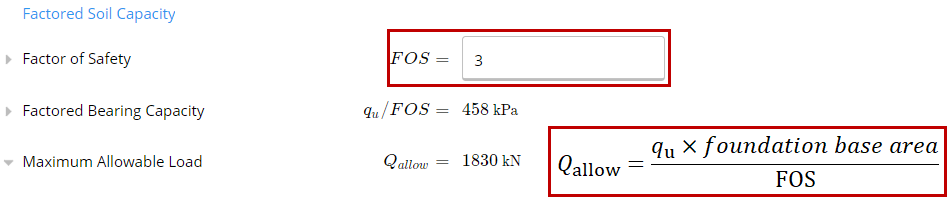## Assumptions and Limitations

• In the present sheet only single soil strata has been considered.
• Water level could be as high as near to ground (H = 0 m) and much below the ground (H > footing depth).
• No lateral load or moment have been considered at anywhere in the calculations.
• In the case of footings in marine environment or heavy loads acting on the footing, case specific design should be carried out.

## References

• B. M. Das (2011) Principles of Foundation Engineering.